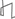Research Abstracts Home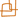CSAIL Digital Archive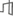Research Activities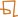CSAIL HomeResearch Abstracts - 2007IntroductionPerception & LearningPhysical, Biological & Social SystemsSystemsTheory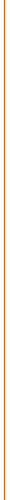### Exponentiated Gradient Algorithms for Log-Linear Structured Prediction

#### Amir Globerson, Xavier Carreras, Terry Koo & Michael Collins

##### Abstract

Conditional log-linear models are a popular method for structured prediction. Efficient learning of the model parameters is therefore an important problem, and becomes a key factor when learning from very large data sets. This paper describes an exponentiated gradient (EG) algorithm for training such models. EG is applied to the convex dual of the maximum likelihood objective; this results in both sequential and parallel update algorithms, where in the sequential algorithm parameters are updated in an online fashion. We provide a convergence proof for both algorithms, which also applies to max-margin learning, simplifying previous results on EG learning of max-margin models, and leading to a tighter bound on the convergence rate for these models. Experimental results on a large scale parsing task show that the proposed algorithm converges much faster than a conjugate-gradient approach both in terms of optimization objective and test error.

##### Overview

Structured learning problems involve the prediction of objects such as sequences or parse trees. The set of possible objects may be exponentially large, but each object typically has rich internal structure. Several models that implement learning in this scenario have been proposed over the last few years [1,2]. The underlying idea in these methods is to classify each instanceinto a label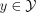using a prediction ruleHereis a feature vector, andis a set of weights that are learned from labeled data. The methods differ in their approach to learning the weight vector. The Maximum Margin Markov Networks of Taskar et al.  rely on margin maximization, while the Conditional Random Fields (CRFs) of Lafferty et al.  construct a conditional log-linear model and maximize the likelihood of the observed data. Empirically both methods have shown promising results on complex structured labeling tasks.

In both CRFs and max-margin models, the learning task involves an optimization problem which is convex, and therefore does not suffer from problems with local minima. However, finding the optimal weight vector w may still be computationally intensive, especially for very large data sets with millions of data points. In the current paper, we propose a fast and efficient algorithm for optimizing log-linear models such as CRFs.

To highlight the difficulty we address, consider the common practice of optimizing the conditional log-likelihood of a CRF using conjugate gradient algorithms, or other methods such as L-BFGS . Any update to the weight vector would require at least one pass over the data set, and typically more due to line-search calls. Since conjugate gradient convergence typically requires several tens of iterations if not hundreds, the above optimization scheme can turn out to be quite slow. It would seem advantageous to have an algorithm that updates the weight vector after every sample point, instead of after the entire data set. One example of such algorithms is stochastic gradient descent and its variants .

A different approach, which we employ here, was first suggested by Jaakkola and Haussler . It proceeds via the convex dual problem of the likelihood maximization problem. As in the dual Support Vector Machine (SVM) problem, the dual variables correspond to data points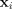. Specifically, to every pointthere corresponds a distribution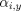satisfying:It is thus tempting to optimizefor eachseparately, much like the Sequential Minimization Optimization (SMO) algorithm  often used to optimize SVMs. Several authors (e.g. Keerthi et al. ) have studied such an approach, using different optimization schemes, and ways of maintaining the distribution constraints on. Here we present an algorithm based on Exponentiated Gradient (EG) updates . These updates are specifically designed for optimizing over distributions, and were recently used in the max-margin setting . Our use of EG results in very simple updates that can be performed for every sample point, giving an online-like algorithm.

We provide a convergence proof of the algorithm, using a symmetrization of the KL divergence. Our proof is relatively simple, and applies both to the max-margin and log-linear settings. Furthermore, we show that convergence can be obtained for the max-margin case with a higher learning rate than that used in the proof of Bartlett et al. , giving a tighter bound on the rate of convergence.

Finally, we compare our EG algorithm to a conjugate gradient optimization on a standard multiclass learning problem, and a complex natural language parsing problem. In both settings we show that EG converges much faster than conjugate gradient, both in terms of objective function and classification error.

##### Primal and Dual Problems for Log-Linear Models

Consider a supervised learning setting where we are given training data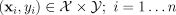. A globally-normalized log-linear model defines the following conditional probability distribution over labels: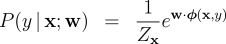where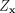is the partition function andis an appropriate feature mapping. We wish to find the weightsthat maximize the regularized log-likelihood, which yields the following convex primal optimization problem: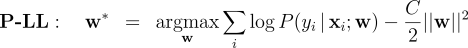where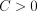is the regularization constant. The above has an equivalent convex dual, as derived by Lebanon & Lafferty . For each example and label, introduce the dual variable, and define vectors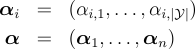as aggregates of these duals. Letbe the set of distributions over the labels for a given example. The dual problem can be expressed aswhere the dual objective function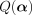is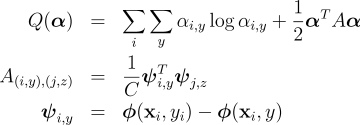The method of Exponentiated Gradient (EG) updates was first introduced by Kivinen and Warmuth  and extended to structured learning by Bartlett et al. . We briefly describe batch and online-like methods for performing EG updates on our dual optimization problem (cf. D-LL above).

Given an initial distribution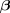and the gradient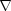of the objective function at that point, the EG update is defined bywhereis a constant learning rate that controls how strongly the algorithm adjusts the parameters. Note that the normalization ofensures that the updated parameters form a distribution. For convenience, define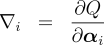The batch and online-like EG updates are then simple to describe. In the batch setting, we fix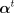, compute all gradients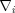and update all of the duals simultaneously via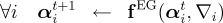In the online setting, we consider each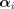in sequence, compute the appropriate gradientand perform the update##### Convergence of the Batch and Online Updates

We briefly sketch our proof of convergence. The analysis relies on manipulations of three divergence measures between sets of distributions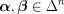: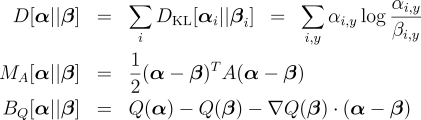Here,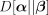is the cumulative KL divergence between the two sets of distributions,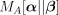is the Mahalanobis distance (parameterized by the matrix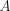), andis the Bregman divergence (parameterized by a strictly convex function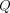). For both log-linear and max-margin definitions of the dual objective, it is possible to obtain a constant learning ratefor which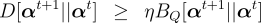By the definition ofand, it follows that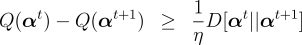This result allows us to deduce that both batch and online-like EG updates will eventually converge at the optimum of the dual problem D-LL. Furthermore, by applying an additional lemma from Kivinen and Warmuth , we can prove that the batch algorithm will converge withinof the optimum afterupdates. Unfortunately, this lemma does not apply to the online-like EG updating strategy, and we have not yet proven a rate of convergence for this strategy. However, our experiments indicate that online-like EG updates converge considerably faster than state-of-the-art batch optimization approaches such as conjugate gradient descent and quasi-Newton methods (e.g., L-BFGS).

##### Acknowledgements

This work was funded by a grant from the National Science Foundation (DMS-0434222) and a grant from NTT, Agmt. Dtd. 6/21/1998. In addition, A. Globerson is supported by a postdoctoral fellowship from the Rothschild Foundation - Yad Hanadiv.

##### References:

 B. Taskar, C. Guestrin, and D. Koller. Max-margin markov networks. In NIPS, 2004.

 J. Lafferty, A. McCallum, and F. Pereira. Conditional random fields: Probabilistic models for segmenting and labeling sequence data. In Proceedings of ICML, 2001.

 Sha, F., and Pereira, F. Shallow parsing with conditional random fields. In Proceedings of HLT-NAACL, 2003.

 Vishwanathan, S. N., Schraudolph, N. N., Schmidt, M.W., and Murphy, K. P. Accelerated training of conditional random fields with stochastic gradient methods. In Proceedings of ICML, 2006.

 Jaakkola, T., and Haussler, D. Probabilistic kernel regression models. In Proceedings of AISTATS, 1999.

 Platt, J. Fast training of support vector machines using sequential minimal optimization. In Advances in kernel methods - support vector learning. MIT Press, 1998.

 Keerthi, S., Duan, K., Shevade, S., and Poo, A. N. A fast dual algorithm for kernel logistic regression. Machine Learning, 61, 151-165, 2005.

 Kivinen, J., and Warmuth, M. Exponentiated gradient versus gradient descent for linear predictors. Information and Computation, 132, 1-63, 1997.

 Bartlett, P., Collins, M., Taskar, B., and McAllester, D. Exponentiated gradient algorithms for large-margin structured classification. In NIPS, 2004.

 Lebanon, G, and Lafferty, J. Boosting and maximum likelihood for exponential models. In NIPS, 2004.Computer Science and Artificial Intelligence Laboratory (CSAIL) The Stata Center, Building 32 - 32 Vassar Street - Cambridge, MA 02139 - USA tel:+1-617-253-0073 - publications@csail.mit.edu